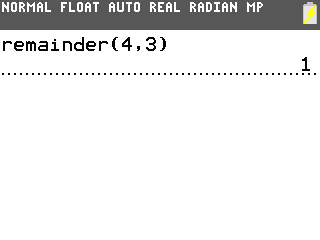# Knowledge Base

## Solution 36757: Calculating Quotient and Remainders on the TI-84 Plus Family of Graphing Calculators.

### When I divide two integers, how can I get the quotient and remainder instead of a decimal answer or an improper fraction on the TI-84 Plus family of graphing calculators?

The TI-84 Plus family of graphing calculators do not have an integer division function that would return the quotient and remainder of a division of integers. However, a combination of the normal division and the remainder( ) function can be used to calculate both the quotient and remainder.

For example, the division 4/3 will return 1.3333333 in Auto mode.  The number of units 1 is the quotient.

To find the remainder follow the step below:

1) Press [MATH] [Right arrow]  to select 0:remainder(
2) Press  [,]  [ ) ] and then press [ENTER].3) 1 is returned, which means the division of 4/3 equals 1 with a remainder of 1 or 1+1/3.